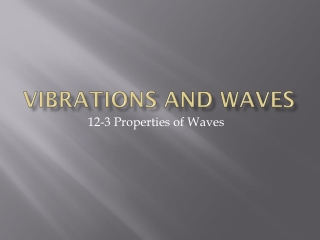DownloadDownload PresentationVibrations and Waves

# Vibrations and Waves

Télécharger la présentation## Vibrations and Waves

- - - - - - - - - - - - - - - - - - - - - - - - - - - E N D - - - - - - - - - - - - - - - - - - - - - - - - - - -
##### Presentation Transcript

1. Vibrations and Waves 12-3 Properties of Waves

2. Medium – material through which a disturbance travels. Ex: water, air Mechanical waves : waves that require a material medium Ex: Sound waves – without air as a medium to travel through, there would be no sound. Wave Motion • A wave is the motion of a disturbance. • Waves of almost every kind require a material medium to travel through. • Waves that require a material medium are called mechanical waves. Figure 12-10 A pebble dropped into a pond creates ripple waves similar to those shown here.

3. Wave Types • A wave that consists of a single traveling pulse is called a pulse wave. • When you have several pulses generated, these pulses form a periodic wave. Figure 12-11 A single flip of a wrist on a taut rope creates a pulse wave.

4. Wave Types • When a waves displacement is perpendicular to its motion, this is called a transverse wave. • When particles vibrate parallel to direction of wave motion, this is called a longitudinal wave.

5. Longitudinal waves • These are also known as Compression Waves. • Compressions – More dense; darker area (crest) • RareFactions – Less dense area (trough)

6. Parts of a Wave • Crest - Highest point above equilibrium. • Trough – Lowest point below equilibrium. • Wavelength – Distance the wave travels during one cycle ().

7. Wave Speed • Wave speed equals frequency times wavelength. Formula = Speed of Wave = frequency x wavelength v = f

8. Waves Transfer Energy • The rate at which waves transfer energy depends on amplitude. • The greater the amplitude, the more energy a wave carries in a given time interval.

9. Guided Practice • Open Books to page 457 • Sample 12D

10. Vibrations and Waves 12-4 Wave Interactions

11. Wave Interference • Two different material objects can never occupy the same space at the same time. • Waves, however, can pass through one another. • When this happens, the waves form an interference pattern. Figure 12-17 This ripple tank demonstrates the interference of water waves.

12. Types of Interference • Constructive interference – displacements on same side of equilibrium. • The method of summing the displacements of waves is known as the superposition principle. Figure 12-18 When these two wave pulses meet, the displacements at each point add up to form a resultant wave. This is an example of constructive interference.

13. Types of Interference • Destructive Interference – displacements on opposite sides of equilibrium. • Complete Destructive Interference – two pulses equal in amplitude but on opposite sides of equilibrium completely cancel each other. Figure 12-19 In this case, known as destructive interference, the displacement of one pulse is subtracted from the displacement of the other.

14. Reflection • At a free boundary, waves are reflected. • At a fixed boundary, waves are reflected and inverted.

15. Standing Waves • Standing waves have nodes and antinodes. • The points at which two waves cancel are nodes • Between two adjacent nodes where the amplitude is the greatest, these points are called anti nodes.